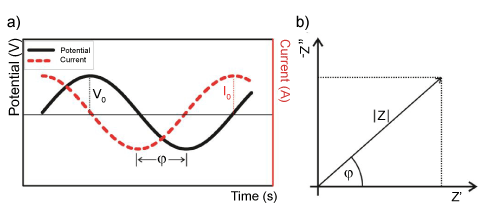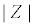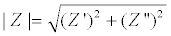Figure 5: a) Time domain measurements of an impedance analysis at a single frequency. A sinusoidal voltage perturbation (amplitude V0) and the sinusoidal current response with amplitude I0 has a phase angle ϕ. (b) The impedance value associated to the data from (a) can be described as the modulus, ϕ and the real Z′ and imaginary Z″ parts, respectively